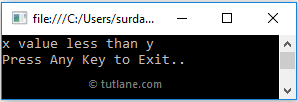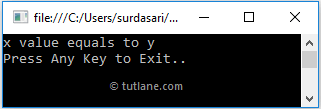# C# Ternary Operator (?:) with Examples

In c#, Ternary Operator (?:) is a decision-making operator, and it is a substitute for the if…else statement in c# programming language.

Using Ternary Operator, we can replace multiple lines of if…else statement code into a single line in c# programming language.

The Ternary operator will help you execute the statements based on the defined conditions using the decision-making operator (?:).

## Syntax of C# Ternary Operator

In c#, the Ternary Operator will always work with 3 operands. Following is the syntax of defining a Ternary Operator in c# programming language.

condition_expression? first_expression : second_expression;

If you observe the above Ternary Operator syntax, the conditional operator (?:) will return only one value from the defined expressions, either first_expression or second_expression based on the value of a condition.

In c#, the Ternary Operator (?:) will work as follow.

• In Ternary Operator, the condition expression must be evaluated to be either true or false. If the condition is true, the first_expression result is returned by the ternary operator.
• In case the condition is false, then the second_expression result is returned by the operator.

As said earlier, the Ternary Operator (?:) is a substitute for the if…else statement in c# programming language. For example, we can replace the following if…else statement with Ternary Operator (?:) like as shown following.

int x = 5, y = 20;
string result;

// if...else statement if (x > y)
{
result = "x greater than y";
}
else {
result = "x less than y";
}

//Ternary Operator (?:) statement
result = (x > y) ? "x greater than y" : "x less than y";

If you observe the above example, we simplified the if…else condition by replacing multiple lines of the if…else condition code with Ternary Operator (?:) in c# programming language.

Now, we will see the complete example of a Ternary operator (?:) in c# programming language.

## C# Ternary Operator Example

Following is the example of using a Ternary Operator (?:) in c# programming language.

using System;

namespace Tutlane
{
class Program
{
static void Main(string[] args)
{
int x = 5, y = 20;
string result;
//Ternary Operator (?:)
result = (x > y) ? "x value greater than y" : "x value less than y";
Console.WriteLine(result);
Console.WriteLine("Press Enter Key to Exit..");
}
}
}

If you observe the above code, we used a Ternary Operator (?:) to evaluate an expression (x > y) to show the result based on our requirements.

When you execute the above c# program, you will get the result below.This is how we can use Ternary Operator (?:) as a substitute for if…else statement in c# programming language.

## C# Nested Ternary Operator

In c#, we can create a Nested Ternary Operator by including multiple conditional expressions as a second or third part of expressions in the ternary operator. These nested ternary operators will help us replace if…else if statements in c# programming language.

Following is the example of replacing if…else if statement with a nested ternary operator in c# programming language.

int x = 20, y = 20;
// If...else If Statement
string result;
if (x > y)
{
result = "x value greater than y";
}
else if (x < y)
{
result = "x value less than y";
}
else {
result = "x value equals to y";
}
//Nested Ternary Operator (?:)
result = (x > y) ? "x value greater than y" : (x < y) ? "x value less than y" : "x value equals to y";

If you observe the above code, we are able to replace multiple lines of if…else if code with a single line of the nested ternary operator based on our requirements.

In c#, the conditional operator is a right-associative so the expression a ? b : c ? d : e; evaluated as a ? b : (c ? d : e), not as (a ? b : c) ? d : e.

## C# Nested Ternary Operator Example

Following is the example of defining a nested ternary operator in the c# programming language.

using System;

namespace Tutlane
{
class Program
{
static void Main(string[] args)
{
int x = 20, y = 20;
string result;
//Nested Ternary Operator (?:)
result = (x > y) ? "x value greater than y" : (x < y) ? "x value less than y" : "x value equals to y";
Console.WriteLine(result);
Console.WriteLine("Press Enter Key to Exit..");
}
}
}

When we execute the above c# program, we will get the result below.This is how we can implement a nested ternary operator in c# programming language to replace if…else if statements based on our requirements.